# RS Aggarwal Solutions for Class 9 Chapter 7: Lines and Angles Exercise 7A

Students often don’t clear their doubts in the class when solved by the teacher owing to shyness. This mainly occurs in a subject like Mathematics because in other subjects the concepts can be understood by re-reading the chapter. But, in Mathematics, the students need to understand the methods which are used in solving problems. In order to help students, we provide exercise wise answers to all the chapters in PDF format. By referring the PDF, students can understand the methods used in solving the problems. RS Aggarwal Solutions for Class 9 Maths Chapter 7 Lines and Angles Exercise 7A are provided here.

## RS Aggarwal Solutions for Class 9 Chapter 7: Lines and Angles Exercise 7A Download PDF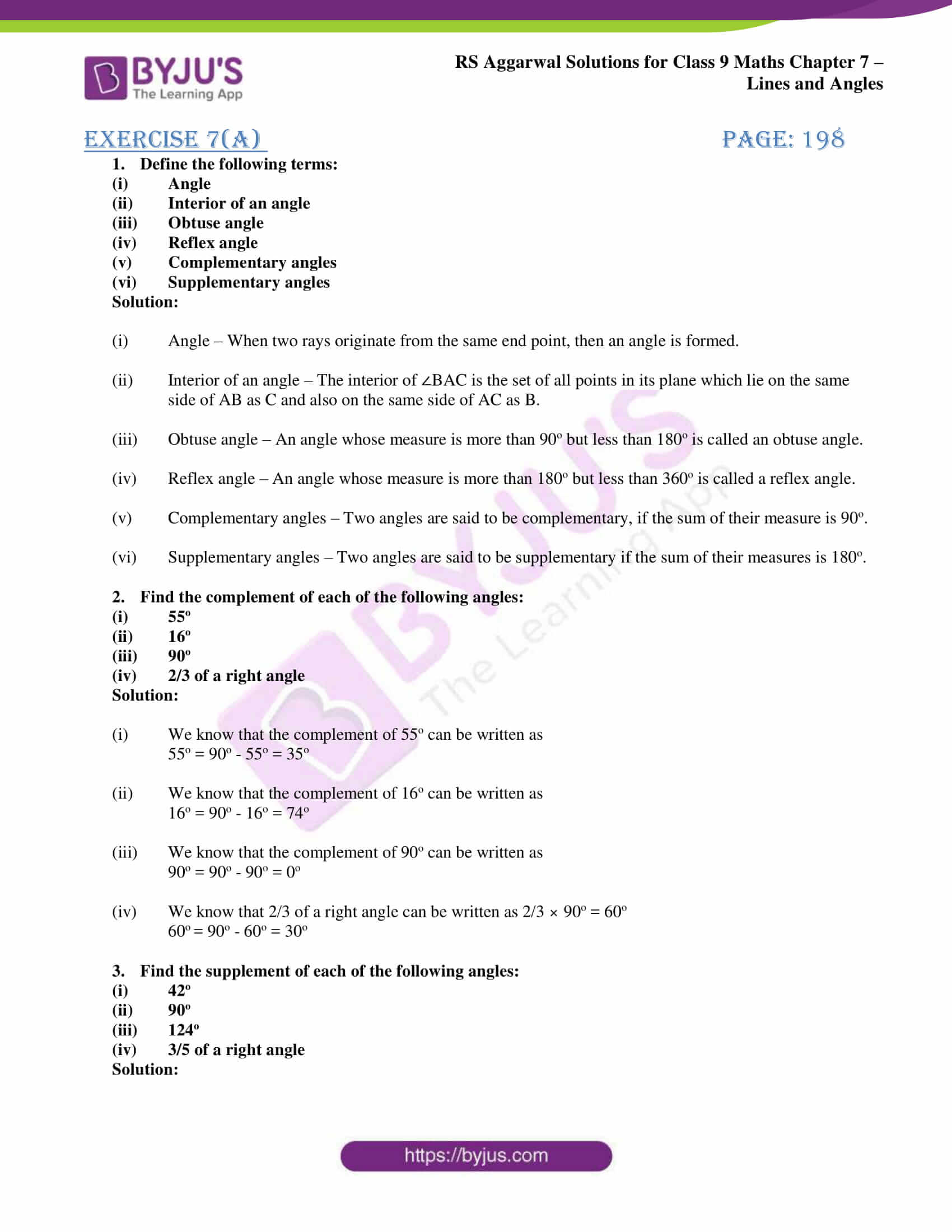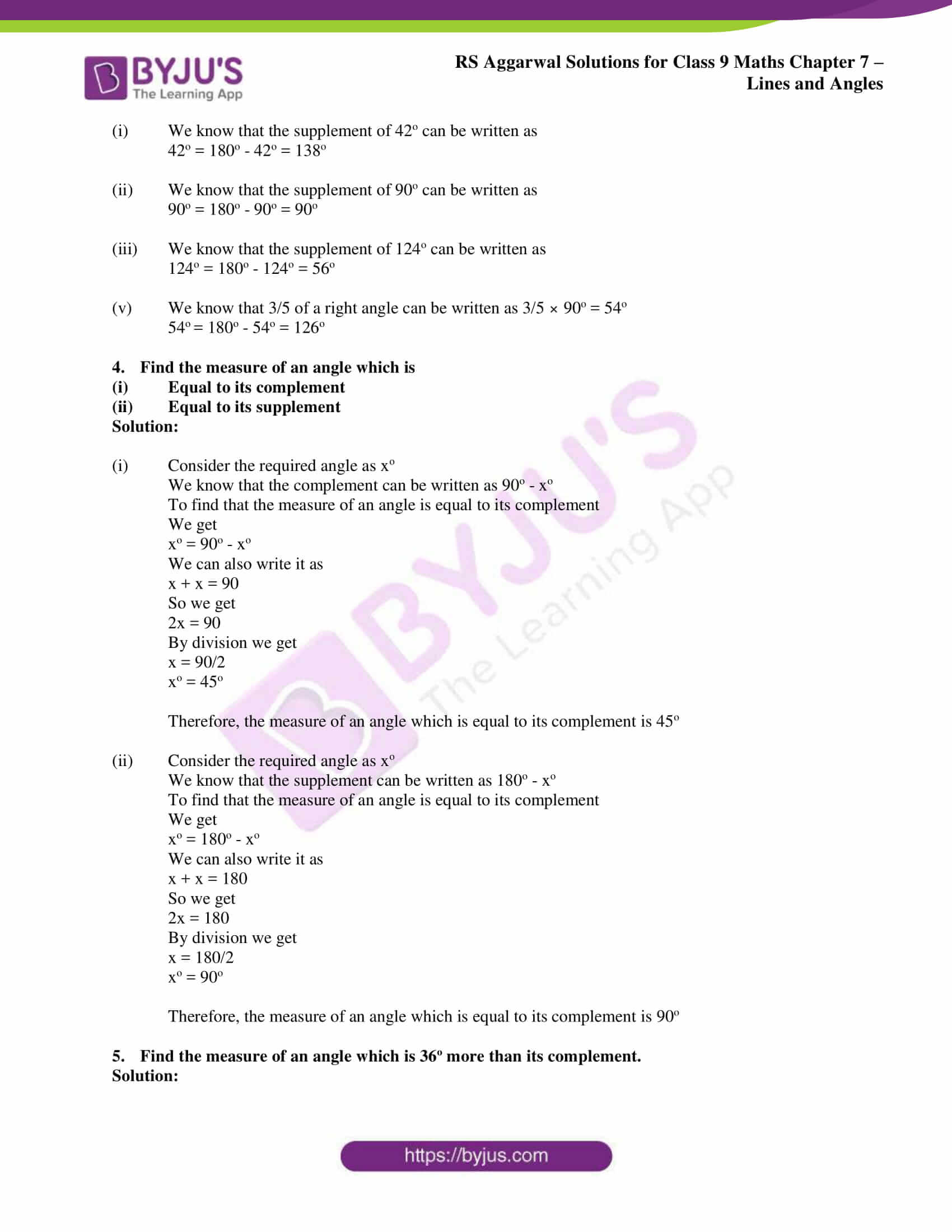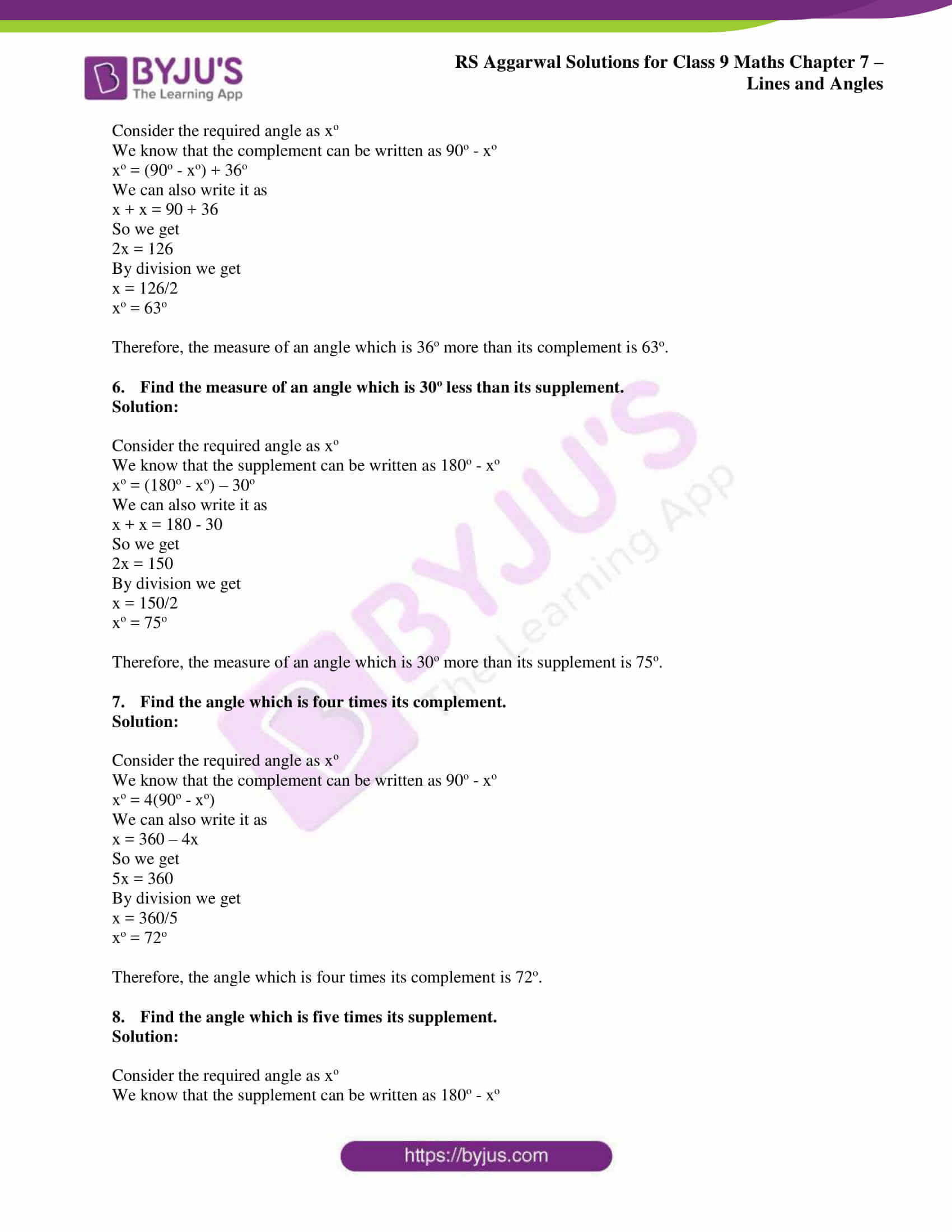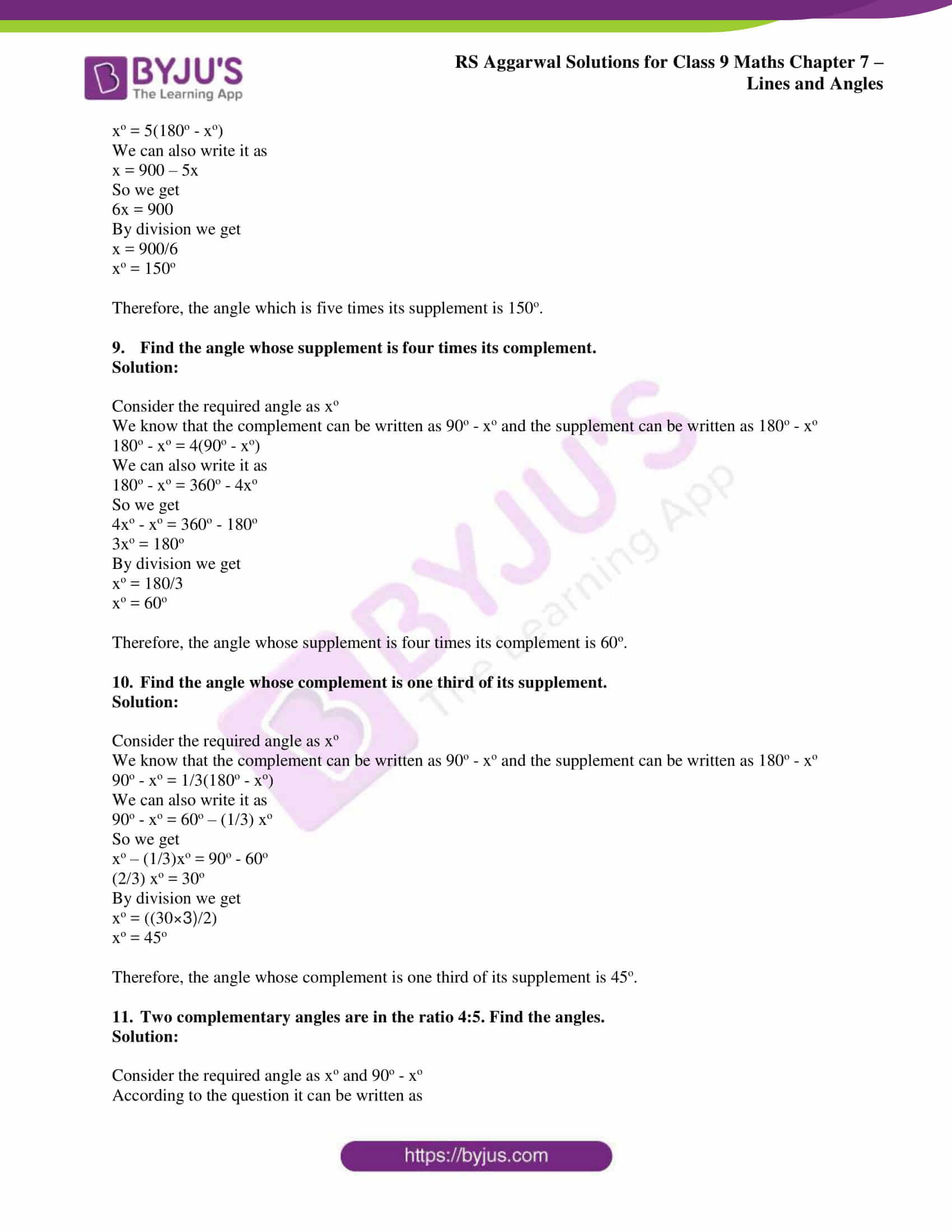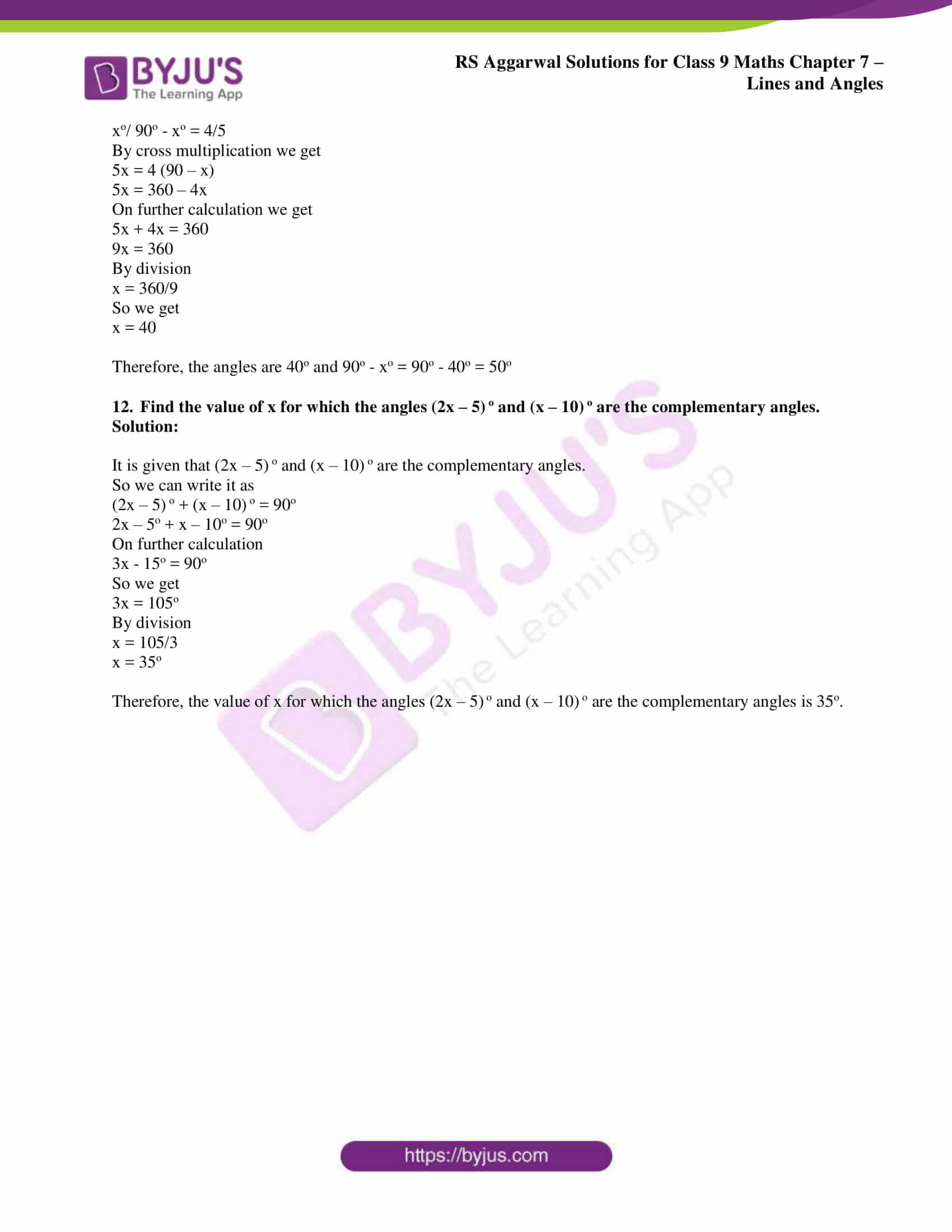## Access RS Aggarwal Solutions for Class 9 Chapter 7: Lines and Angles Exercise 7A

Exercise 7(A)

1. Define the following terms:

(i) Angle

(ii) Interior of an angle

(iii) Obtuse angle

(iv) Reflex angle

(v) Complementary angles

(vi) Supplementary angles

Solution:

(i) Angle – When two rays originate from the same end point, then an angle is formed.

(ii) Interior of an angle – The interior of ∠BAC is the set of all points in its plane which lie on the same side of AB as C and also on the same side of AC as B.

(iii)Obtuse angle – An angle whose measure is more than 90o but less than 180o is called an obtuse angle.

(iv) Reflex angle – An angle whose measure is more than 180o but less than 360o is called a reflex angle.

(v) Complementary angles – Two angles are said to be complementary, if the sum of their measure is 90o.

(vi) Supplementary angles – Two angles are said to be supplementary if the sum of their measures is 180o.

2. Find the complement of each of the following angles:

(i) 55o

(ii) 16o

(iii) 90o

(iv) 2/3 of a right angle

Solution:

(i) We know that the complement of 55o can be written as

55o = 90o – 55o = 35o

(ii) We know that the complement of 16o can be written as

16o = 90o – 16o = 74o

(iii) We know that the complement of 90o can be written as

90o = 90o – 90o = 0o

(iv) We know that 2/3 of a right angle can be written as 2/3 × 90o = 60o

60o = 90o – 60o = 30o

3. Find the supplement of each of the following angles:

(i) 42o

(ii) 90o

(iii) 124o

(iv) 3/5 of a right angle

Solution:

(i) We know that the supplement of 42o can be written as

42o = 180o – 42o = 138o

(ii) We know that the supplement of 90o can be written as

90o = 180o – 90o = 90o

(iii) We know that the supplement of 124o can be written as

124o = 180o – 124o = 56o

(iv) We know that 3/5 of a right angle can be written as 3/5 × 90o = 54o

54o = 180o – 54o = 126o

4. Find the measure of an angle which is

(i) Equal to its complement

(ii) Equal to its supplement

Solution:

(i) Consider the required angle as xo

We know that the complement can be written as 90o – xo

To find that the measure of an angle is equal to its complement

We get

xo = 90o – xo

We can also write it as

x + x = 90

So we get

2x = 90

By division we get

x = 90/2

xo = 45o

Therefore, the measure of an angle which is equal to its complement is 45o

(ii) Consider the required angle as xo

We know that the supplement can be written as 180o – xo

To find that the measure of an angle is equal to its complement

We get

xo = 180o – xo

We can also write it as

x + x = 180

So we get

2x = 180

By division we get

x = 180/2

xo = 90o

Therefore, the measure of an angle which is equal to its complement is 90o

5. Find the measure of an angle which is 36o more than its complement.

Solution:

Consider the required angle as xo

We know that the complement can be written as 90o – xo

xo = (90o – xo) + 36o

We can also write it as

x + x = 90 + 36

So we get

2x = 126

By division we get

x = 126/2

xo = 63o

Therefore, the measure of an angle which is 36o more than its complement is 63o.

6. Find the measure of an angle which is 30o less than its supplement.

Solution:

Consider the required angle as xo

We know that the supplement can be written as 180o – xo

xo = (180o – xo) – 30o

We can also write it as

x + x = 180 – 30

So we get

2x = 150

By division we get

x = 150/2

xo = 75o

Therefore, the measure of an angle which is 30o more than its supplement is 75o.

7. Find the angle which is four times its complement.

Solution:

Consider the required angle as xo

We know that the complement can be written as 90o – xo

xo = 4(90o – xo)

We can also write it as

x = 360 – 4x

So we get

5x = 360

By division we get

x = 360/5

xo = 72o

Therefore, the angle which is four times its complement is 72o.

8. Find the angle which is five times its supplement.

Solution:

Consider the required angle as xo

We know that the supplement can be written as 180o – xo

xo = 5(180o – xo)

We can also write it as

x = 900 – 5x

So we get

6x = 900

By division we get

x = 900/6

xo = 150o

Therefore, the angle which is five times its supplement is 150o.

9. Find the angle whose supplement is four times its complement.

Solution:

Consider the required angle as xo

We know that the complement can be written as 90o – xo and the supplement can be written as 180o – xo

180o – xo = 4(90o – xo)

We can also write it as

180o – xo = 360o – 4xo

So we get

4xo – xo = 360o – 180o

3xo = 180o

By division we get

xo = 180/3

xo = 60o

Therefore, the angle whose supplement is four times its complement is 60o.

10. Find the angle whose complement is one third of its supplement.

Solution:

Consider the required angle as xo

We know that the complement can be written as 90o – xo and the supplement can be written as 180o – xo

90o – xo = 1/3(180o – xo)

We can also write it as

90o – xo = 60o – (1/3) xo

So we get

xo – (1/3)xo = 90o – 60o

(2/3) xo = 30o

By division we get

xo = ((30×3)/2)

xo = 45o

Therefore, the angle whose complement is one third of its supplement is 45o.

11. Two complementary angles are in the ratio 4:5. Find the angles.

Solution:

Consider the required angle as xo and 90o – xo

According to the question it can be written as

xo/ 90o – xo = 4/5

By cross multiplication we get

5x = 4 (90 – x)

5x = 360 – 4x

On further calculation we get

5x + 4x = 360

9x = 360

By division

x = 360/9

So we get

x = 40

Therefore, the angles are 40o and 90o – xo = 90o – 40o = 50o

12. Find the value of x for which the angles (2x – 5) o and (x – 10) o are the complementary angles.

Solution:

It is given that (2x – 5) o and (x – 10) o are the complementary angles.

So we can write it as

(2x – 5) o + (x – 10) o = 90o

2x – 5o + x – 10o = 90o

On further calculation

3x – 15o = 90o

So we get

3x = 105o

By division

x = 105/3

x = 35o

Therefore, the value of x for which the angles (2x – 5) o and (x – 10) o are the complementary angles is 35o.

### Access other exercise solutions of Class 9 Maths Chapter 7: Lines and Angles

Exercise 7B Solutions 16 Questions

Exercise 7C Solutions 24 Questions

### RS Aggarwal Solutions Class 9 Maths Chapter 7 – Lines and Angles Exercise 7A

RS Aggarwal Solutions Class 9 Maths Chapter 7 Lines and Angles Exercise 7A is the first exercise which contains examples and problems which are solved by experts based on complementary and supplementary angles.

### Key features of RS Aggarwal Solutions for Class 9 Maths Chapter 7: Lines and Angles Exercise 7A

• The solutions are prepared by experts keeping in mind the understanding abilities among the students.
• Self analysis can be done by the students to understand the areas of higher importance based on weightage in the exam.
• RS Aggarwal solutions contain answers in descriptive manner which help students grasp the concepts faster.
• The problems are solved using the easiest methods and diagrammatic representation helps students to understand the methods.